# Symmetric Waves

## by Greg EganIn a comment to a blog post I wrote about spherical harmonics, Gerard Westendorp asked an interesting question: Can an atom have dodecahedral symmetry?

My first hunch about this was that the answer would be: only in the sense that you can give an atom’s wave function any shape at all. Spherical harmonics, which describe the angular part of the variation of a hydrogen atom’s wave function, can be used to synthesise any sufficiently well-behaved function on a sphere, but as with Fourier analysis, to get exactly the shape you want might take an infinite number of terms! Each spherical harmonic is characterised by two quantum numbers, ℓ and m, which are associated, respectively, with the magnitude of a particle’s angular momentum and its component measured along some chosen axis. In a hydrogen atom, the value of ℓ must be less than that of a third quantum number, n, that determines the total energy of the particle. If you needed arbitrarily high values of ℓ, you would also need arbitrarily high values of n, and if you used more than a single value of n then the associated waves would oscillate at different rates and slip out of phase with each other over time. What’s more, as Matt McIrvin pointed out, the radial part of the wave function depends on both ℓ and n, so that even for a fixed n, if you needed to use more than a single value for ℓ the angular form of the wave function would not be the same on spheres of different radii.

But happily, my hunch was completely wrong: it’s actually possible to write down wave functions for a hydrogen atom with perfect dodecahedral symmetry using a single value for ℓ (along with any choice of n > ℓ), so that the shape is both constant in time and the same on spheres of all radii. The trick is that you have to choose ℓ from a certain sequence of allowed values, which obey a pattern whose details and origin will be explained below.

(Caveat: Everything that follows assumes that the Schrödinger equation for the hydrogen atom is exactly correct, which of course it isn’t, since it neglects the spin of the electron and all relativistic and quantum field-theoretical corrections that, in reality, give an additional fine structure to the electron wave functions.)

The following write-up is the product of a long discussion on the topic that developed in the comments of another post. John Baez wrote about the results here, and Layra Idarani wrote about them as well. But the three of us have taken somewhat different approaches, and targeted audiences with different mathematical backgrounds.

## Solid Harmonics and Spherical Harmonics

Spherical harmonics are functions on the sphere that describe the angular variation of any quantum wave function for a single particle subject to a radially symmetric potential. But the nicest way to get a handle on them, mathematically, is to talk about functions defined, not just on the sphere, but on all of three-dimensional space.

The functions we will begin with are the homogeneous polynomials in the three Cartesian coordinates, x, y and z. A polynomial is homogeneous if the sum of the powers of all the variables in every term is the same. So, x2 y + x y z + z3 is a homogeneous polynomial in x, y and z of degree 3. The homogeneous polynomials of a given degree form a vector space: adding two such polynomials together, or multiplying them by a constant factor, gives you another such polynomial.

There is an obvious way to “rotate” a homogeneous polynomial and get another one of the same degree. If we imagine the function values “painted” on three-dimensional space, we can rotate this pattern as a rigid object and then describe it again as a new function, and it will once again be a homogeneous polynomial of the same degree. To take a simple example, consider the degree-1 polynomial x+y+z. If we rotate this by 90 degrees counterclockwise around the z-axis, we will get another degree-1 polynomial, yx+z.

To make this work in general, we need a function ρd which maps the group of rotations, SO(3), to the group of linear operators on the vector space of homogeneous polynomials of degree d. For any rotation R, we will define the linear operator ρd(R) by specifying the result of applying it to any particular polynomial, p:

d(R) p)(x) = p(R–1 x)

Using the inverse of the rotation, R–1, might seem a bit strange at first, but this is done to give the nice result that, for any two rotations R and S in SO(3):

ρd(R) ρd(S) = ρd(RS)

A vector space V, like our space of homogeneous polynomials, along with a function like ρd that maps some group G to the group of linear operators on V, is known as a representation of G. So we say that the space of homogeneous polynomials in three variables provides a representation of the group of rotations in three dimensions, SO(3).

We can find the dimension of the vector space of homogeneous polynomials in x, y and z of degree d by first considering all the choices we have for the power of x, which we’ll call dx. This ranges from 0 to d, and in each case the power of y can range from 0 to ddx, while the power of z is completely fixed by the other two choices. So the total number of possibilities is equal to the sum of (ddx+1) as dx ranges from 0 to d, which comes to (d+1)(d+2)/2. So for d=2, say, we will have a vector space of dimension 6, with a basis given by: {x2, xy, xz, y2, yz, z2}.

Now, for d > 1 the representation of SO(3) we get from rotating homogeneous polynomials is reducible: there are subspaces U of this space of polynomials that are left unchanged by ρd. That is to say, ρd(R) can take a polynomial p in U to another polynomial in U, but never to one outside it. We call such a subspace invariant. One obvious example for d=2 is the subspace of all polynomials that are multiples of:

P(x, y, z) = x2 + y2 + z2

This is just the squared distance from the origin, so applying any rotation to it won’t change it at all.

If we want to find irreducible representations of SO(3), we need to impose a further restriction. We require that our polynomials are harmonic, which in this context means they satisfy Laplace’s equation:

2 p = ∂x2p + ∂y2p + ∂z2p = 0

It isn’t hard to see that if we take a solution of Laplace’s equation and rotate it with ρd(R), we will get another solution of Laplace’s equation. So this requirement will single out an invariant subspace of the homogeneous polynomials of any degree. Although we won’t prove it, the subspace of harmonic, homogeneous polynomials of a given degree form an irreducible representation of SO(3), containing no smaller, non-trivial invariant subspace. The representation of SO(3) on the harmonic, homogeneous polynomials of degree d is known as the spin-d representation.

We can find the dimension of the spin-d representation easily enough. If we apply the Laplacian operator, ∇2, to any homogeneous polynomial of degree d we will get another homogeneous polynomial, this time of degree d–2. Requiring this second polynomial to be zero means imposing a number of constraints equal to the dimension of the space of homogeneous polynomials of degree d–2, and so the dimension of the remaining subspace will be:

dim(spin-d) = (d+1)(d+2)/2 – (d–1)d/2 = 2d+1

For d=0 and d=1 all the homogeneous polynomials are automatically harmonic, with bases {1} and {x, y, z} respectively. But for d=2, where the space of homogeneous polynomials is 6-dimensional, the harmonic, spin-2 polynomials form a 5-dimensional subspace, spanned by the five functions {xy, yz, xz, x2y2, x2z2}, while the rest of the space will be spanned by {x2+y2+z2}.

In general, along with the 2d+1 harmonic polynomials of degree d, there will be (d–1)d/2 non-harmonic polynomials of the same degree. What can we say about them? It turns out that if we consider all polynomials of the form:

(x2 + y2 + z2)k Hd–2k(x, y, z)

where k takes on all non-zero values such that d–2k is non-negative, and Hd–2k(x, y, z) is any harmonic homogeneous polynomial of degree d–2k, we can account for all the non-harmonic polynomials of degree d. So these non-harmonic polynomials are just the products of various powers of (x2+y2+z2), and harmonic polynomials of lower degree.

What does this have to do with spherical harmonics? The spherical harmonics associated with the quantum number ℓ are the (2ℓ+1)-dimensional space of functions on the unit sphere that are obtained by evaluating the spin-ℓ harmonic polynomials on that sphere. The harmonic polynomials themselves, defined on all of three-dimensional space, are called solid harmonics.

It is common practice to define a basis for the spin-ℓ spherical harmonics {Yℓ,m}, labelled by the quantum number m, which specifies the component of a particle’s angular momentum pointing along the z-axis, and which ranges from –ℓ to ℓ. The corresponding solid harmonics Sℓ,m are related to the spherical harmonics by the equation:

Sℓ,m = r Yℓ,m

where r is the distance from the origin of the coordinate system. We can write the condition satisfied by the basis elements as:

i (xySℓ,myxSℓ,m) = m Sℓ,m

Here the left-hand side is the operator that measures the z-component of angular momentum, acting on Sℓ,m, and the equation says that Sℓ,m is an eigenfunction of that operator with eigenvalue m. A basis for the solid harmonics with ℓ=2 and m=–2,–1,0,1,2 is:

S2,–2 = ¼ √[15/(2π)] (x2y2 – 2ixy)
S2,–1 = ½ √[15/(2π)] (xiy) z
S2,0 = ¼ √[5/π] (x2 + y2 – 2z2)
S2,1 = ½ √[15/(2π)] (x + iy) z
S2,2 = ¼ √[15/(2π)] (x2y2 + 2ixy)

These functions are all mutually orthogonal (with respect to an inner product defined as the integral over the unit sphere of the product of one function and the complex conjugate of the other), and their inner products with themselves are all 1.

## Dodecahedral Wave Functions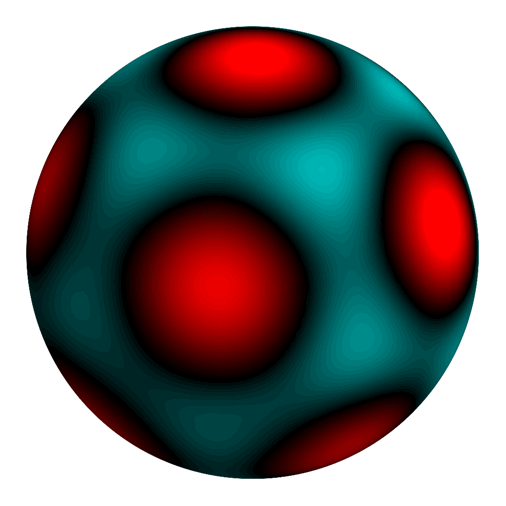Spherical harmonic of degree 6, with the 120 symmetries of a dodecahedron: (√7 Y6,–5 + √(11) Y6,0 – √7 Y6,5)/5Spherical harmonic of degree 10, with the 120 symmetries of a dodecahedron: (√(187/3) Y10,–10 – √(209) Y10,–5 + √(247/3) Y10,0 + √(209) Y10,5 + √(187/3) Y10,10)/25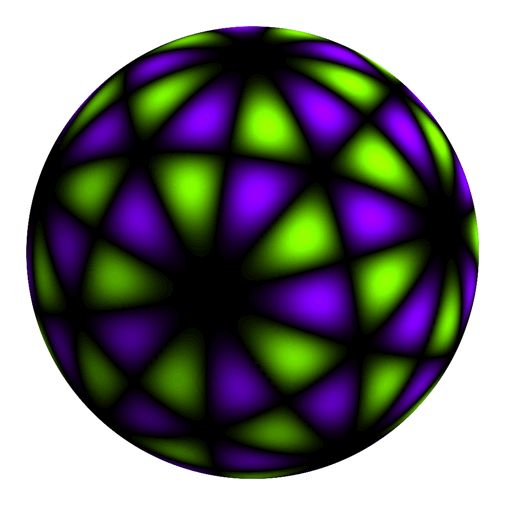Spherical harmonic of degree 15, with the 60 rotational symmetries of a dodecahedron: (√(1001/5) Y15,–15 + 2 √(957/10) Y15,–10 – √(667) Y15,–5 – √(667) Y15,5 – 2 √(957/10) Y15,10 + √(1001/5) Y15,15)/50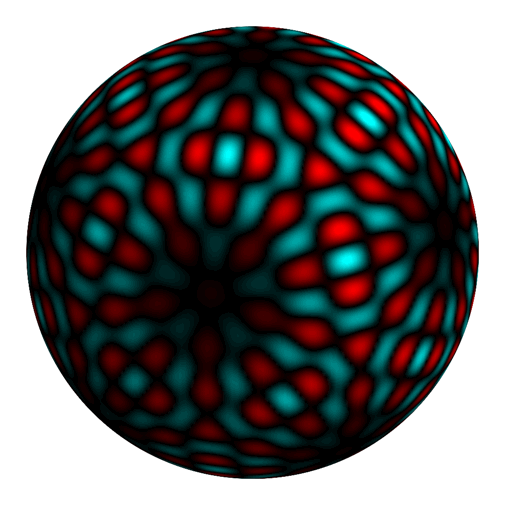Oscillating between two different spherical harmonics of degree 30, both with the 120 symmetries of a dodecahedron.

Any regular dodecahedron is left unchanged by a group of 60 rotations. The exact choice of rotations with respect to the coordinate system will depend on the exact orientation of the dodecahedron, but the different 60-element subgroups of SO(3) obtained this way are all isomorphic to each other, and the rotational symmetries of a suitably oriented icosahedron will be the same. It is traditional to call this group I: the icosahedral group.

If we want to find a spin-ℓ spherical harmonic that is left unchanged by the same symmetries, we can try a simple formula:

M = sum over all R in I of ρ(R)

where by ρ(R) we mean the (2ℓ+1)×(2ℓ+1) matrix that acts on the basis of spherical harmonics, {Yℓ,m}, to rotate all these functions by the rotation R. The matrix M might turn out to be zero, which will mean we’re out of luck with our choice of ℓ, or it might turn out to have a 1-dimensional (or 2- or more-dimensional) range, in which case any vector in its range, construed as a linear combination of the Yℓ,m, will be a spin-ℓ spherical harmonic with the symmetries we desire.

It turns out that the first few values of ℓ for which M is non-zero are 0, 6, 10 and 15. The first case, ℓ=0, is trivial: it gives a constant function on the sphere, which is certainly unchanged by any of the rotations in I, but is rather boring. The other three cases, ℓ=6, 10 and 15, are shown in the images on the left. Here the brightness of each point indicates the magnitude of the function, while the two colours (which are chosen arbitrarily) indicate positive and negative values. Although the individual spherical harmonics, Yℓ,m, are generally complex-valued, these symmetric combinations of them always turn out to be real-valued (or, depending on what conventions are adopted, to have a single complex phase as an overall factor, which can be divided out without changing the symmetries of the function). In all of these images, the rotation is meant as a rotation of the point of view, in order to show more of the function; it is not meant to imply that the wave function itself is physically rotating with the passage of time.

For ℓ=30, the range of M is two-dimensional, so there are two orthogonal spin-30 functions with dodecahedral symmetry. The bottom image on the left oscillates between these two possibilities. As with the rotation, this oscillation is not intended to portray a physical effect, but merely to provide a way of illustrating the full range of possibilities.

The rank of M turns out to obey a relatively simple rule: it is always equal to the number of distinct ways (without regard to order) that ℓ can be written as a sum of the numbers 6, 10 and 15, with the restriction that 15 appears at most once in any such sum.

This is zero for:

ℓ = 1, 2, 3, 4, 5, 7, 8, 9, 11, 13, 14, 17, 19, 23, 29,

one for:

ℓ = 0, 6, 10, 12, 15, 16, 18, 20, 21, 22, 24, 25, 26, 27, 28, 31, 32, 33, 34, 35, 37, 38, 39, 41, 43, 44, 47, 49, 53, 59,

two for:

ℓ = 30, 36, 40, 42, 45, 46, 48, 50, 51, 52, 54, 55, 56, 57, 58, 61, 62, 63, 64, 65, 67, 68, 69, 71, 73, 74, 77, 79, 83, 89,

and so on, adding 30 to the previous list of ℓ values to find the examples with the next highest rank.

Where does this rule come from? The easiest way to understand it is probably through a result of Claude Chevalley, who described all the polynomials that are invariant under a related, but larger symmetry group, known to crystallographers as Ih and mathematicians as H3, which consists of all rotations and products of rotations and reflections that preserve an icosahedron or dodecahedron. This larger group has 120 elements, compared to the 60 elements in I.

Chevalley showed that all polynomials invariant under H3 can be written as linear combinations of products of the following three polynomials, of degree 2, 6 and 10:

P(x, y, z) = x2 + y2 + z2
Q(x, y, z) = product over i=1,..,6 of (x, y, z) · vi
R(x, y, z) = product over i=1,..,10 of (x, y, z) · fi

where v1, ..., v6 are any choice of six vertex-axes of the icosahedron (that is, six vectors that are parallel to the lines joining the six antipodal pairs of vertices), and f1, ..., f10 are any choice of ten face-centre-axes of the icosahedron, similarly defined. These vectors can have any non-zero length and point in either direction along the axes without changing any of the properties we’re interested in, so those choices are really just a matter of convenience.

Obviously P will be invariant under all rotations and reflections, while any symmetry of the icosahedron will map vertices to vertices and face-centres to face-centres, so the polynomials Q and R will at most change sign. In fact they are entirely invariant. The easiest way to see this is to note that the planes through the origin orthogonal to either the vertex-axes or the face-centre-axes (which contain all the points for which these polynomials are zero) are not planes of mirror symmetry for the icosahedron. So in the neighbourhood of a generic point that does lie on a plane of symmetry, Q and R will not change sign from one side of the plane to the other. That means they cannot change sign under any of the elements of H3 that are pure reflections, and since those elements generate the whole group, they cannot change sign at all.

Chevalley also showed that P, Q and R are not connected by any non-trivial relations. What that means is that if we take any set of polynomials of the form {Pi Qj Rk} for distinct triplets of exponents (i, j, k), the set will be linearly independent: no non-trivial linear combination of these polynomials will be identically zero. In particular, if we choose all the triplets such that 2i+6j+10k=d, then the set {Pi Qj Rk} will give us a basis for all homogeneous polynomials of degree d that are invariant under H3.

Because we are interested in polynomials that are invariant under I but not necessarily under the larger group H3, we will also make use of one more polynomial:

T(x, y, z) = product over i=1,..,15 of (x, y, z) · ei

where e1, ..., e15 are any choice of fifteen edge-centre-axes of the icosahedron. In this case, T will change sign under some elements of H3, but is invariant under the smaller group I of rotations only. To see this, we again consider the planes of symmetry of the icosahedron, which turn out to coincide exactly with the planes through the origin orthogonal to the edge-centre-axes. In the neighbourhood of a generic point on such a plane, T will change sign across the plane. So it will change sign under any of the pure reflections in H3, and any product of an odd number of these reflections, which are precisely the elements of H3 that are not in I.

The pattern we are trying to explain is that the number of linearly independent harmonic homogeneous polynomials of degree ℓ that are invariant under I is equal to the number of ways that ℓ can be written as a sum of 6, 10 and 15, with 15 used at most once. The result of Chevalley, plus our additional polynomial T, would seem to suggest that if we dropped the requirement that the polynomials are harmonic, we ought to count the ways ℓ can be written as a sum of 2, 6, 10 and 15, with 15 used at most once. The need to use 15 at most once is not too mysterious: if we square T, we will get a polynomial that is invariant under all of H3, so by Chevalley it must be possible to write T2 in terms of P, Q and R, which would make any appearance of more than one factor of T redundant.

The question, then, is how we connect all this to the case when the polynomials are required to be harmonic. We saw earlier that the space of homogeneous polynomials of any given degree splits up into a subspace of polynomials with no overall factor of P, which gives the harmonic polynomials, and then subspaces with various powers of P as a factor, all of which are non-harmonic. If we form a polynomial p as a product of powers of Q, R and T, then it will have no overall factor of P. That does not guarantee that p itself is harmonic, because it might be the sum of a harmonic polynomial and a non-harmonic polynomial. But it does guarantee that, when p is written as a sum of terms from each of the subspaces with different powers of P as factors, it will include a non-zero term that is not divisible by P at all, and is thus harmonic.

So, the number of independent polynomials of a given degree that we can form as a product of powers of Q, R and T (with T used at most once) will give us the correct count of the number of harmonic polynomials of the same degree that are invariant under I, because although the product itself need not be harmonic, it will have a component in the subspace of harmonic polynomials. If two such products had the same harmonic component, then their difference would have to be divisible by P, but since neither is divisible by P individually, that would imply some relation between P, Q and R, which Chevalley ruled out.

While P and Q have harmonic components, they are not themselves harmonic. But T is a pure harmonic! This turns out to be part of a general pattern: for every symmetry group, there is a polynomial like this that is invariant under the group of rotations, but changes sign under reflections, and which is harmonic.

Why harmonic? The Laplacian operator ∇2 commutes with rotations and reflections, so ∇2 T must also be invariant under rotations while changing sign under reflections. That means ∇2 T must either be zero, or a polynomial of a lower degree with the same symmetries as T. If it was non-zero, we could keep taking the Laplacian repeatedly, until we arrived at a polynomial of the lowest degree among all polynomials with the same symmetries, and whose Laplacian was zero.

In fact, T itself has the lowest possible degree of all polynomials that change sign under reflections, since it has zeroes on the mirror planes of all the pure reflections in H3, and nowhere else. So T, and the analogous functions for other symmetry groups, must be harmonic.

## Tetrahedral Wave FunctionsSpherical harmonic of degree 6, with the 12 rotational symmetries of a tetrahedron: (√(5/2) Y6,–6 – √(11/2) Y6,–2 – √(11/2) Y6,2 + √(5/2) Y6,6)/4Oscillating between two different spherical harmonics of degree 6, one with the 12 rotational symmetries of a tetrahedron, the other with all 24 symmetries.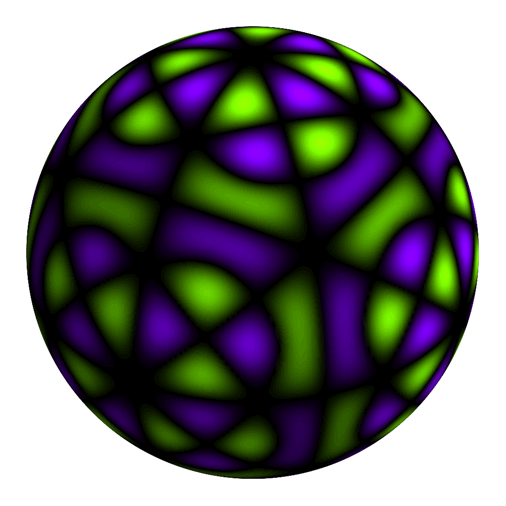Oscillating between two different spherical harmonics of degree 17, one with the 12 rotational symmetries of a tetrahedron, the other with all 24 symmetries.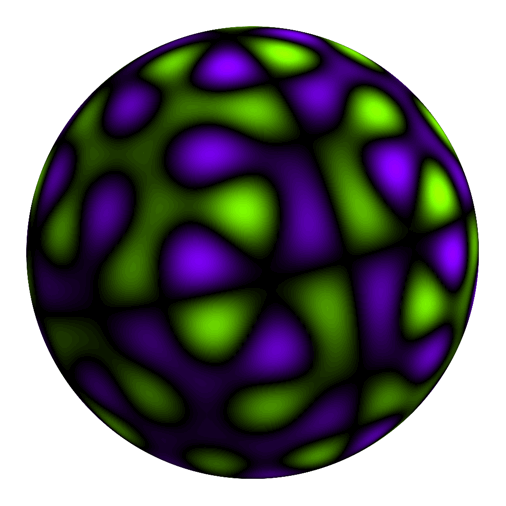Oscillating between two different spherical harmonics of degree 15, one with the 12 rotational symmetries of a tetrahedron, the other with the 60 rotational symmetries of a dodecahedron.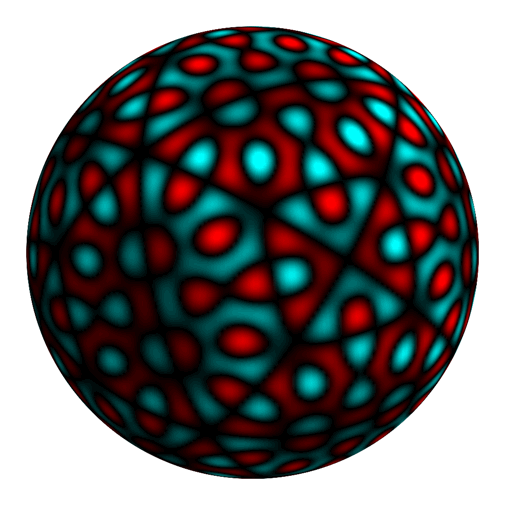Oscillating between two different spherical harmonics of degree 30, one with 24 symmetries (the 12 rotational symmetries of a tetrahedron, plus inversion), the other with all of the 120 symmetries of a dodecahedron.

We can use the same general approach to create wave functions that share the rotational symmetry group, O, of the cube and octahedron, or T, the rotational symmetry group of the tetrahedron. As before, we sum the matrices for the spin-ℓ representation over the elements of the symmetry group, and if the sum M is non-zero then any vectors that lie in its range will describe harmonic polynomials with the desired symmetries.

There are also analogous versions of the Chevalley results we described for the dodecahedron / icosahedron. For the cube, we start by defining some polynomials by multiplying together dot products with axes that pass through various features. If we use a cube whose faces are aligned with the coordinate axes, we have:

A(x, y, z) = (xyz) (x+yz) (xy+z) (x+y+z)
From the cube’s 4 vertex-axes, an invariant of degree 4.
B(x, y, z) = (xy) (x+y) (xz) (x+z) (yz) (y+z)
From the cube’s 6 edge-centre-axes, a semi-invariant (a function that changes sign under some rotations) of degree 6.
C(x, y, z) = x y z
From the cube’s 3 face-centre-axes, a semi-invariant of degree 3.

From these, we can construct a set of invariants for O:

A of degree 4 (also an invariant of the group Oh, which includes reflections).
C2 of degree 6 (also an invariant of Oh).
BC of degree 9 (which is harmonic, and not an invariant of Oh). This is the lowest-degree polynomial that is zero on all the planes of mirror symmetry of the cube.

B2 can be expressed in terms of A, C2 and P (and its harmonic component can be expressed without P), so we never use it, and we use BC at most once. So the number of independent polynomials in spin ℓ with O as their symmetry group is the number of ways to write ℓ as a sum of 4, 6 and at most one 9.

For the tetrahedron, to make life simpler we can choose four vertices of the cube to be the vertices of our tetrahedron. We then have the following invariants of T, which are all functions we have already defined:

C of degree 3 (also an invariant of the group Th, which includes reflections).
A of degree 4 (also an invariant of the group Th).
B of degree 6 (which is harmonic, and not an invariant of Th). This is the lowest-degree polynomial that is zero on all the planes of mirror symmetry of the tetrahedron.

Since B2 can be expressed in terms of A, C2 and P, we use B at most once. So the number of independent polynomials in spin ℓ with T as their symmetry group is the number of ways to write ℓ as a sum of 3, 4 and at most one 6.

There are two independent tetrahedrally-symmetric wave functions of degree 6: one is just the degree-6 polynomial B (which is itself harmonic), while the other is the harmonic component of C2. The first image on the right shows B, while the second image oscillates between B and the harmonic component of C2.

The third image on the right oscillates between two tetrahedrally-symmetric wave functions of degree 17, derived from the two ways we can write 17 as 3+4+4+6 and 3+3+3+4+4. So these are the harmonic components of CA2B and C3A2.

The last two images on the right oscillate between pairs of harmonic polynomials of the same degree that have different symmetry groups: tetrahedral and dodecahedral ... and in this case the latter have been built from the former. How? You can rotate five copies of a regular tetrahedron so that all 20 vertices end up coinciding with the vertices of a regular dodecahedron: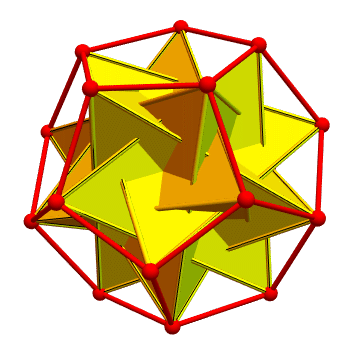If we perform the same kind of rotations on five copies of a wave function with tetrahedral symmetry and add up the results, we will get a wave function with dodecahedral symmetry. The images shown oscillate between a sum like that and the wave function that was used to build the sum, for two different choices of the original tetrahedral wave function, one with degree 15, and one with degree 30. For the degree 15 case, the tetrahedral wave function possesses no extra symmetries: its complete symmetry group is T. As a result, the dodecahedral sum is chiral, like the compound of 5 tetrahedra itself: it is not the same as its mirror image.

For the degree 30 case, the tetrahedral wave function does not have any of the reflection symmetries of a tetrahedron, but it is symmetric under inversion: replacing (x, y, z) with (–x, –y, –z). The effect of that extra symmetry is to wash out the chiral nature of the compound, and the dodecahedral sum ends up being mirror-symmetric.

## Four-dimensional Harmonics

All of the techniques we used to find harmonic polynomials that shared the symmetry groups of three-dimensional polyhedra can be applied in four dimensions, to construct harmonic polynomials in four variables that share the symmetry groups of four-dimensional polytopes.

The largest such symmetry group is known as H4, and it contains the 14,400 symmetries (both rotations and products of rotations and reflections) of the 600-cell and the 120-cell. These two polytopes are, in a sense, the four-dimensional analogues of the icosahedron and dodecahedron; the first has 600 tetrahedral cells (“cells” being the three-dimensional parts of a polytope that are the analogue of the two-dimensional faces of a polyhedron), while the second has 120 dodecahedral cells. If we wish to consider only rotational symmetries, these polytopes have 7,200 of them.

The lowest degrees for which harmonic polynomials exist with the rotational symmetries of a 600-cell are: 0, 12, 20, 24, 30, 32, 36, 40, 42, 44, 48, 50, 52, 54, 56 and 60. As before, this pattern can be explained by considering Chevalley polynomials, with degrees 12, 20, 30 and 60, with the degree-60 polynomial used at most once.

The degree-60 polynomial can be constructed as the product of dot products with the 60 vertex-axes of the 600-cell (which has 120 vertices). These vertex-axes are also the normals to all the hyperplanes of mirror symmetry of the 600-cell. As with the three-dimensional examples, this polynomial changes sign under reflection, and it is harmonic.

The lower-degree Chevalley polynomials are a bit trickier to construct. If we consider a 120-cell, each of its cells is a dodecahedron. For a single dodecahedron, we know how to construct three-dimensional invariants of degree 6, 10 and 15, and we could do this for one dodecahedral cell, taking products of dot products with the axes that pass between pairs of vertices, face-centres and edge-centres. If we then used the 7,200 rotations in H4 to rotate the original choice of axes, and added up all the rotated functions, we should end up with invariants of degrees 6, 10 and 15. However, the sum of the rotated polynomials turns out to be zero in all three cases.

Luckily, there is a simple remedy: we just square the functions before we rotate them, giving polynomials of degrees 12, 20 and 30 that are invariant under the 7,200 rotations (and in fact, invariant under all of H4). These polynomials are not harmonic, but they do have non-zero harmonic components.

The images below, on the left, show four-dimensional harmonic polynomials of degrees 12, 20, 30 and 60 with the symmetries of a 600-cell. To portray these four-dimensional functions, we take an ordinary sphere in three dimensions (mathematicians call this a 2-sphere, since the spherical surface itself is two-dimensional), and rotate it so that, over time, it sweeps out a 3-sphere in four dimensions. At each instant, the image shows the values of the function on the rotated 2-sphere.

In the case of three-dimensional harmonic polynomials, each such polynomial could be thought of as describing the angular variation of a possible wave function for a hydrogen atom with well-defined values for the electron’s energy and the magnitude of its angular momentum. The radial variation would then be given by an additional, non-polynomial function related to the quantum numbers ℓ and n. Remarkably, though, any four-dimensional harmonic polynomial can be used to construct a complete wave function for a hydrogen atom, again with a well-defined energy, but this time built up from multiple components with different values for ℓ. The resulting three-dimensional wave function will only share the full group of symmetries of the four-dimensional harmonic in an abstract sense, but will have some spatial symmetries that form a subgroup of the four-dimensional symmetry group.

The images below, on the right, show the three-dimensional wave functions associated with the four-dimensional harmonic polynomials beside them. The precise connection between the two kinds of function takes some explaining: the short version is that we stereographically project the four-dimensional harmonic from the surface of a 3-sphere into three-dimensional momentum space, and then transform it from momentum space into ordinary space. Much more detail can be found in this survey of the subject, “The Ellipse and the Atom”.

The three-dimensional wave functions shown are all invariant under the group I of rotational symmetries of an icosahedron, with the first three invariant under all of Ih = H3 (just as the corresponding four-dimensional functions are invariant under all of H4), while the last function changes sign under reflections, again matching the behaviour of the four-dimensional version. This, along with the value for n in each case, tells us which values of ℓ can be present in components of the wave function: in the first three cases, ℓ must be an even number in the set of degrees compatible with invariance under I, while in the last case, it must be an odd number from the same set.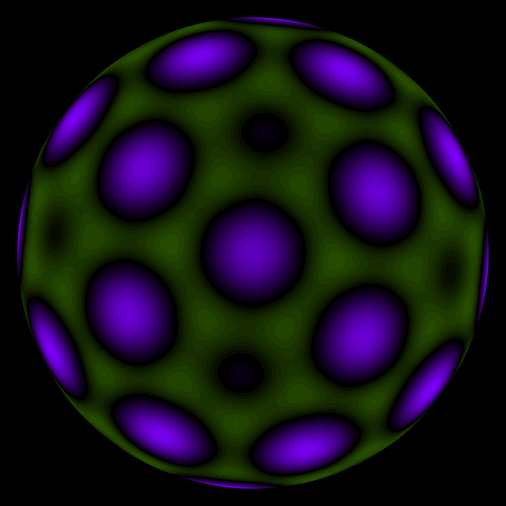4D spherical harmonic of degree 12, with the 14,400 symmetries of a 600-cell. Hydrogen wave function for n=13 (and ℓ = 0, 6, 10, 12), derived from the degree 12 harmonic.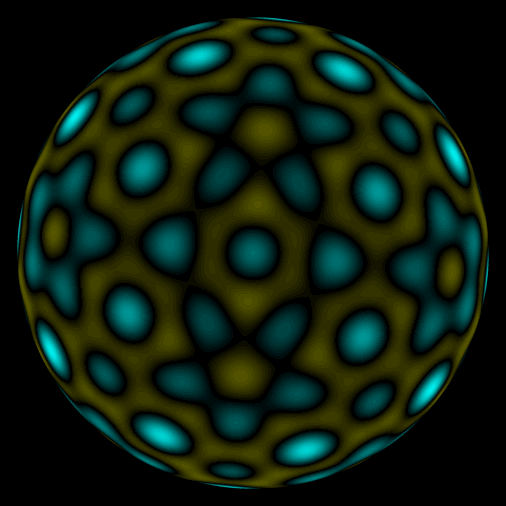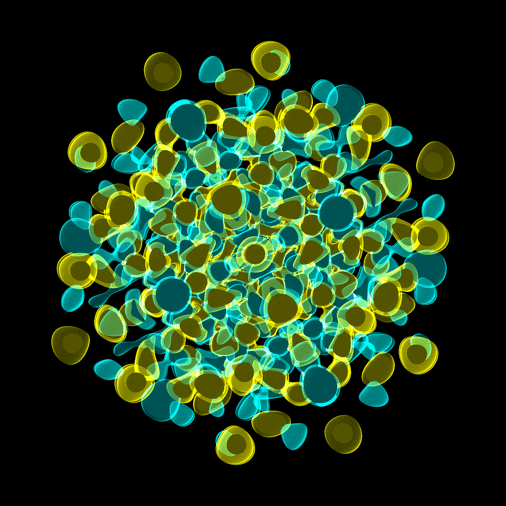4D spherical harmonic of degree 20, with the 14,400 symmetries of a 600-cell. Hydrogen wave function for n=21 (and ℓ = 0, 6, 10, 12, 16, 18, 20), derived from the degree 20 harmonic.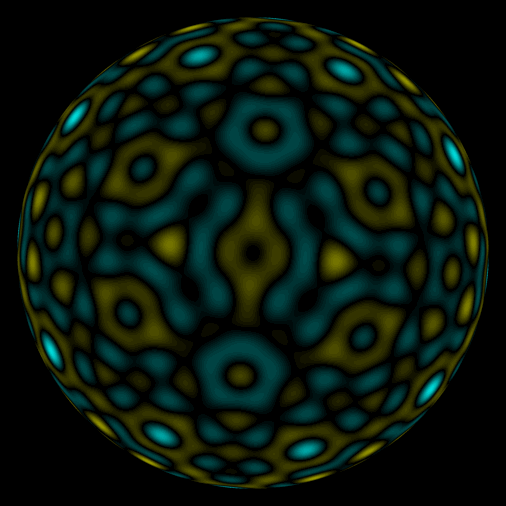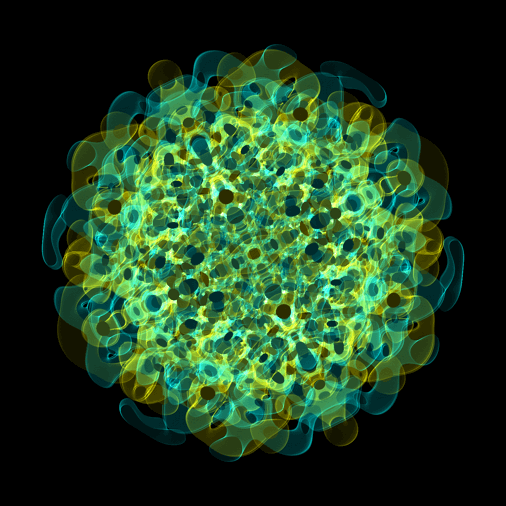4D spherical harmonic of degree 30, with the 14,400 symmetries of a 600-cell. Hydrogen wave function for n=31 (and ℓ = 0, 6, 10, 12, 16, ..[even].., 30), derived from the degree 30 harmonic.4D spherical harmonic of degree 60, with the 7,200 rotational symmetries of a 600-cell. Hydrogen wave function for n=61 (and ℓ = 15, 21, 25, 27, 31, ..[odd].., 59), derived from the degree 60 harmonic.

## References

 Claude Chevalley, “Invariants of finite groups generated by reflections”. Amer. J. Math. 77 (4): 778–782 (1955). doi:10.2307/2372597Science Notes / Symmetric Waves / created Wednesday, 7 February 2018